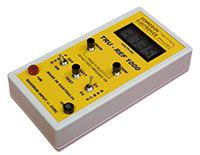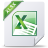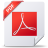#### TRU-REF High Impedance MeterThe monitoring of reference potentials in concrete or dry sand can be significantly influenced by cell loading using a standard 10 megohm voltmeter. This is because reference cells in concrete applications have been seen to be particularly susceptible to error due to having a high source impedance.

The TRU-REF has the ability to overcome this problem by measuring the cell voltage using input impedances of much greater than 10 megohm. Also, by comparing the voltage at two known input impedances (e.g. 10M and 1000M ohm) the true potential and cell source impedance can be calculated.

##### What is Source Voltage and Source Impedance ?

Source voltage or true potential can be described as the reference cell voltage that would be measured if the voltmeter doing the measuring had an infinite input impedance (i.e. an ideal voltmeter). Whereas source impedance refers to the amount of resistance in the current path of the reference cell being measured. Generally speaking, the higher the source impedance, the more inaccurate the readings become when measuring with a standard voltmeter. Calculating the source impedance can offer a great way to quantify a reference cells reliability when compared to other reference cells.

On this webpage you will find an excel spreadsheet which can calculate the source voltage and source impedance based on the readings taken from 2 different and known input impedances.

##### Features:
• Hand-held and lightweight
• Easy to use and read
• Highly Accurate
• Dual Range ±2 V / ±5 V
• 10/100/1000 MΩ Input Impedance
• Battery test function
• Dimensions: 170 x 85 x 35 mm